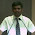## Sunday, March 6

### Arrays in QTP - Part 2

In the 1st part of this post we discussed about Static(fixed size) arrays. Now we are going to discuss another type of array - Dynamic Array.

Dynamic Arrays:-

A dynamic array, also called resizable array is a variable-size array that allows elements to be added or removed at runtime. Dynamic arrays are useful when size of the array cannot be determined at the time of declaration.

Dynamic or Variable Sized Array
2.1. Single dimentional Dynamic Array
2.2. Multi-dimentional Dynamic Array

Dim myArray()  'create a dynamic array.

It creates an array, which can be resized at runtime. For initial use, it must be initialized with some value i.e. size

This will be done using ReDim.

ReDim myArray(3)   'before using first time

Now you can assign values to array

myArray(0)=43
myArray(1)=90
myArray(2)=34
myArray(3)=76

Dynamic array can be resized n number of times, but remember, if you resize it again using ReDim, all its previous data will lost.
For that purpose, Preserve is used with ReDim.

ReDim Preserve myArray(5)

myArray(4)=67
myArray(5)=82

ReDim Preserve resize the array by keeping existing data safe!!

Dynamic arrays can be Multidimensional but only one of the dimensions (the 'right side') can be changed. Refer the below code..

Dim myArray()
Redim myArray(1,0)
myArray(0,0) = "John"
myArray(1,0) = 21
msgbox (ubound (myArray, 2)) '0
Redim preserve myArray(ubound (myArray, 1), ubound (myArray, 2) + 1)
myArray(0,1) = "Bob"
myArray(1,1) = 21
msgbox (ubound (myArray, 2)) '1

So point to be noted about Dynamic Arrays:-

(i) -   Dynamic Arrays are variable-size arrays that allows elements to be added or removed at runtime

(ii) -  Dynamic Arrays are useful when size of the array cannot be determined at declaration time

(iii) - Dynamic Arrays must be initialized with some value i.e. size before using first time

(iv) - Dynamic Arrays can be resized n number of time

(v) - 'Preserve' must be used with ReDim to keep the existing data of Dynamic Arrays

In case of any queries, please post your comments. Have a nice QTPing :)

#### 11 comments:

1.Hi Abhikansh,
I have a doubt here. When we initially declare arrays using Dim MyArray(), we cant differentiate between static and dynamic array. Its only when we use ReDim My Array(3),we can tell that the above declaration{Dim MyArray()} was for dynamic arrays.Am i correct?

2.Dim MyArray()

see the above statement.. there is no numeric value in (). It clearly demonstrates that it’s a dynamic array. In case of Static array, there will a value in parenthesis, i.e Dim MyArray(3)

3.Right..Thanks alot!!

4.good example
thanks :)

5.Hi Abhikansh Jain,

I have a string
str=(mahesh,noor,noor,mahesh,kather,arun,meeran ,arun,kather,ka,k)

We have to print only the unique values for example we have to print
str=(mahesh,noor,kather,arun,meeran,ka,k)

Could you tell me the code

Thanks,
Kather

6.@Kather,

Refer following link..

http://www.qtpschool.com/2011/12/find-distinct-unique-values-from-array.html?utm_source=BP_recent

7.Nice blog, I learn all about dynamic Arrays,, Keep It Bro !!1

8.Hi, I have a doubt..how to store multiple datas in a single variable at runtime using commas which is dynamic may be there will be increase in values? Like Ex: want to store a data as "samplevar= 1,2,3,4,5,6"

9.Hi Vimal,
this below code will do.
Dim i : i = 1
Dim strRepatString : strRepatString = ""
Dim temp
For i = 1 To 10 Step 1
temp = strRepatString&i
strRepatString = temp&","
Next
Msgbox temp

10.str="mahesh,noor,noor,mahesh,kather,arun,meeran ,arun,kather,ka,k"
arr=split(str,",")
for i=Lbound(arr) to Ubound(arr)

x=instr(str,arr(i))
y=instrRev(str,arr(i))

if x=y then
Print "************unique Value**********"
str3=str3&arr(i)
end if
Next
print str3

11.How to declare 5 dimensional array